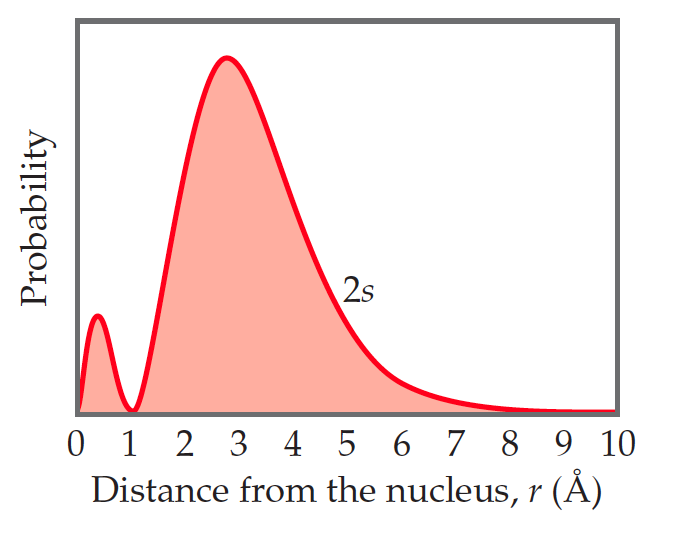# Problem: You may want to reference (Pages 228 - 231) Section 6.5 while completing this problem.The radial probability function for a 2s orbital is shown here.Classify the following statements as either true or false.(a) There are two maxima in this function because one electron spends most of its time at an approximate distance of 0.5 Å from the nucleus and the other electron spends most of its time at an approximate distance of 3 Å from the nucleus.(b) The radial probability function shown here and the probability density [Ψ(r)]2 both go to zero at the same distance from the nucleus, approximately 1 Å.(c) For an s orbital, the number of radial nodes is equal to the principal quantum number, n.

###### Problem Details

You may want to reference (Pages 228 - 231) Section 6.5 while completing this problem.

The radial probability function for a 2s orbital is shown here.Classify the following statements as either true or false.
(a) There are two maxima in this function because one electron spends most of its time at an approximate distance of 0.5 Å from the nucleus and the other electron spends most of its time at an approximate distance of 3 Å from the nucleus.
(b) The radial probability function shown here and the probability density [Ψ(r)]2 both go to zero at the same distance from the nucleus, approximately 1 Å.
(c) For an s orbital, the number of radial nodes is equal to the principal quantum number, n.